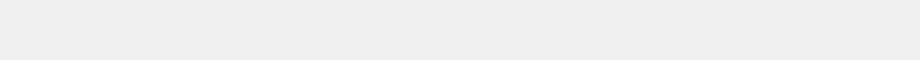Random Real Numbers
Random real numbers are floating point numbers with a uniform distribution. The numbers have a 64-Bit floating point resolution which has a 52-Bit mantissa accounting for the exponent and sign bits. The random numbers from the generator are normalized [-1.0, 1.0]. The API can scale the numbers to any values.
Random Real Numbers
Minimum Value and Maximum Value sets the scaling range for the random numbers. These values can be any valid real number.
How Many Numbers is the Total count of numbers. Example, 2 columns of 5 numbers, is a total of 10 numbers. Maximum count is 1,048,576 (2^20).
How Many Columns arranges the numbers into the specified number of columns.
No HTML suppresses sending HTML wrapper codes. The HTML codes enable proper display of the numbers on a web browser. HTML codes are never used when the numbers are sent as a file.
Send as Text File sends the numbers as a text file instead of sending as a web page. If the total count of numbers is greater than 16384 then the numbers are always sent as a file.
Space Separated Columns have a single space characters between numbers in a row.
Comma Separated Columns
have a comma instead of a space character separating columns.
Quoted Comma Separated columns are separated by a comma and the numbers are surrounded by the double quotes character.
JSON Array returns the numbers with the following JSON format:

{"result":[
numbers here
]}

JSON Array Quoted Numbers is the same as JSON Array but each number is surrounded by the double quotes character.